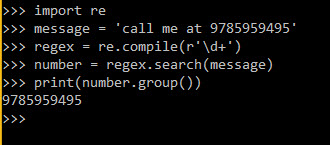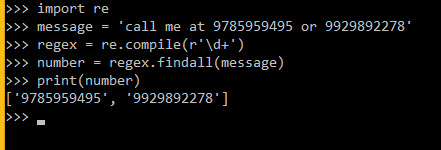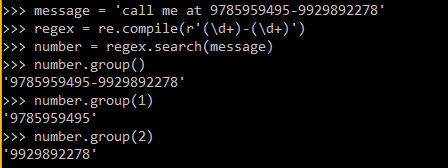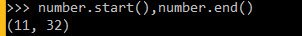# Python Regular Expression

## Introduction

In the chapter, we will learn to use Regular Expression in Python.

## Regular Expression

A regular expression is a particular series of characters that allows you to fit or find certain strings or string sets, utilizing a different syntax in a script.

Syntax
• \s — White space characters
• \S — Non-white space characters
• \w — Word characters
• \W — Non-word characters
• \d — Digit characters
• \D — Non-digit characters
Methods and Attributes for search characters
• match() - Determine if the RE matches at the beginning of the string.
• search() - Scan through a string, looking for any location where this RE matches.
• findall()- Find all substrings where the RE matches and returns them as a list.
• finditer()- Find all substrings where the RE matches and returns them as an iterator.
Methods and Attributes for match object instances
• group() Return the string matched by the RE
• start() Return the starting position of the match
• end() Return the ending position of the match
• span() Return a tuple containing the (start, end) positions of the match
1. Now I’m giving you some examples of regular expressions in Python.
1. import re
2. message = 'call me at 9785959495'
3. regex = re.compile(r'\d+')
4. number = regex.search(message)
5. print(number.group())
Output: 9785959495According to the above Example show library re is useful for finding numbers in the message. r’\d+’ here r denotes this is a line and r does not perform escape conversion like \d and \d means search digits and + means total digits.

2. Getting All digits from particular statements
1. import re
2. message = 'call me at 9785959495 or 9929892278'
3. regex = re.compile(r'\d+')
4. number = regex.findall(message)
5. print(number)
Output : ['9785959495', '9929892278']3. Getting in multiple groups value
1. message = 'call me at 9785959495-9929892278'
2. regex = re.compile(r'(\d+)-(\d+)')
3. number = regex.search(message)
4. number.group()
Output : '9785959495-9929892278'
1. number.group(1
Output: 9785959495
1. number.group(2
Output: 9929892278Python has many methods for regular expression techniques to find or search characters in regular expression library.

## Conclusion

In the next chapte, we will learn how we can use database with the help of Python.
Next » Python Database Handling